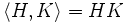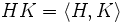# Nilpotent join of pronormal subgroups is pronormal

## Statement

Suppose$H, K \le G$ are Pronormal subgroup (?)s, such that$\langle H, K \rangle$ is a Nilpotent group (?). Then:

•$H$ and$K$ normalize each other.
•$\langle H,K \rangle = HK$ is also a pronormal subgroup of$G$.

## Proof

Given:$H, K \le G$ are pronormal subgroups, and$\langle H, K \rangle$ is nilpotent.

To prove:$H$ and$K$ normalize each other, and$HK = \langle H, K \rangle$ is also pronormal.

Proof: By fact (1),$H,K$ are both pronormal in$\langle H, K \rangle$. By fact (2), they are also both subnormal in$\langle H, K \rangle$. By fact (3),$H, K$ are both normal in$\langle H, K$. Thus, we have$HK = \langle H, K \rangle$, and that$H$ and$K$ normalize each other. By fact (4), we obtain that$\langle H, K \rangle = HK$ is also pronormal.